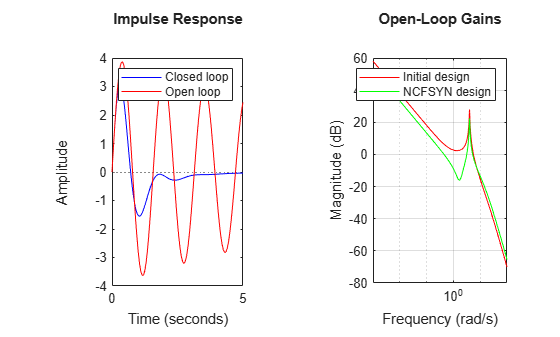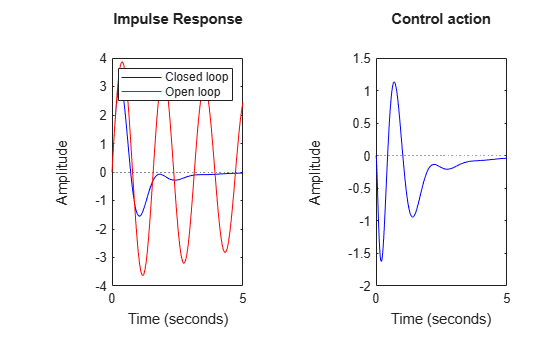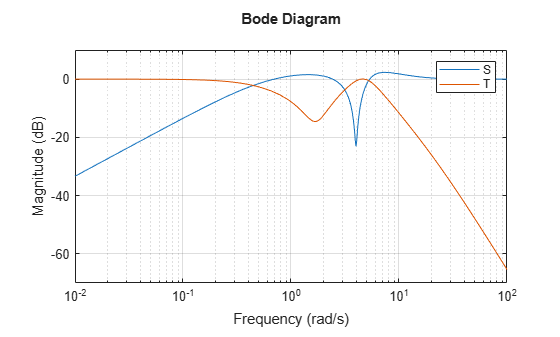# Loop Shaping Using the Glover-McFarlane Method

This example shows how to use `ncfsyn` to shape the open-loop response while enforcing stability and maximizing robustness. `ncfsyn` measures robustness in terms of the normalized coprime stability margin computed by `ncfmargin`.

### Plant Model

The plant model is a lightly damped, second-order system.

$P\left(s\right)=\frac{16}{{s}^{2}+0.16s+16}$.

A Bode plot shows the resonant peak.

```P = tf(16,[1 0.16 16]); bode(P)```### Design Objectives and Initial Compensator Design

The design objectives for the closed-loop are the following.

• Integral action and a bandwidth of at least 0.5 rad/s

• Gain crossover frequencies no larger than 7 rad/s

In loop-shaping control design, you translate these requirements into a desired shape for the open-loop gain and seek a compensator that enforces this shape. For example, a compensator consisting of a PI term in series with a high-frequency lag component achieves the desired loop shape.

```K_PI = pid(1,0.8); K_rolloff = tf(1,[1/20 1]); Kprop = K_PI*K_rolloff; bodemag(P*Kprop); grid```Unfortunately, the compensator `Kprop` does not stabilize the closed-loop system. Examining the closed-loop dynamics shows poles in the right half-plane.

`pole(feedback(P*Kprop,1))`
```ans = 4×1 complex -20.6975 + 0.0000i 0.4702 + 5.5210i 0.4702 - 5.5210i -0.4029 + 0.0000i ```

### Enforcing Stability and Robustness with `ncfsyn`

You can use `ncfsyn` to enforce stability and adequate stability margins without significantly altering the loop shape. Use the initial design `Kprop` as loop-shaping pre-filter. `ncfsyn` assumes a positive feedback control system (see `ncfsyn`), so flip the sign of `Kprop` and of the returned controller.

```[K,~,gamma] = ncfsyn(P,-Kprop); K = -K; % flip sign back gamma```
```gamma = 1.9885 ```

A value of the performance `gamma` less than 3 indicates success (modest gain degradation along with acceptable robustness margins). The new compensator `K` stabilizes the plant and has good stability margins.

`allmargin(P*K)`
```ans = struct with fields: GainMargin: [6.3267 11.1183] GMFrequency: [1.6110 15.1526] PhaseMargin: [80.0229 -99.6598 63.7949] PMFrequency: [0.4471 3.1461 5.2318] DelayMargin: [3.1238 1.4443 0.2128] DMFrequency: [0.4471 3.1461 5.2318] Stable: 1 ```

With `gamma` approximately 2, the expect at most `20*log10(gamma)` = 6dB gain reduction in the high-gain region and at most 6dB gain increase in the low-gain region. The Bode magnitude plot confirms this. Note that `ncfsyn` modifies the loop shape mostly around the gain crossover to achieve stability and robustness.

```subplot(1,2,1) bodemag(Kprop,'r',K,'g',{1e-2,1e4}); grid legend('Initial design','NCFSYN design') title('Controller Gains') subplot(1,2,2) bodemag(P*Kprop,'r',P*K,'g',{1e-3,1e2}); grid legend('Initial design','NCFSYN design') title('Open-Loop Gains')```Figure 1: Compensator and open-loop gains.

### Impulse Response

With the `ncfsyn` compensator, an impulse disturbance at the plant input is damped out in a few seconds. Compare this response to the uncompensated plant response.

```subplot(1,2,1) impulse(feedback(P,K),'b',P,'r',5); legend('Closed loop','Open loop')``````subplot(1,2,2); impulse(-feedback(K*P,1),'b',5) title('Control action')```Figure 2: Response to impulse at plant input.

### Sensitivity Functions

The closed-loop sensitivity and complementary sensitivity functions show the desired sensitivity reduction and high-frequency noise attenuation expressed in the closed-loop performance objectives.

```S = feedback(1,P*K); T = 1-S; clf bodemag(S,T,{1e-2,1e2}), grid legend('S','T')```### Conclusion

In this example, you used the function `ncfsyn` to adjust a hand-shaped compensator to achieve closed-loop stability while approximately preserving the desired loop shape.